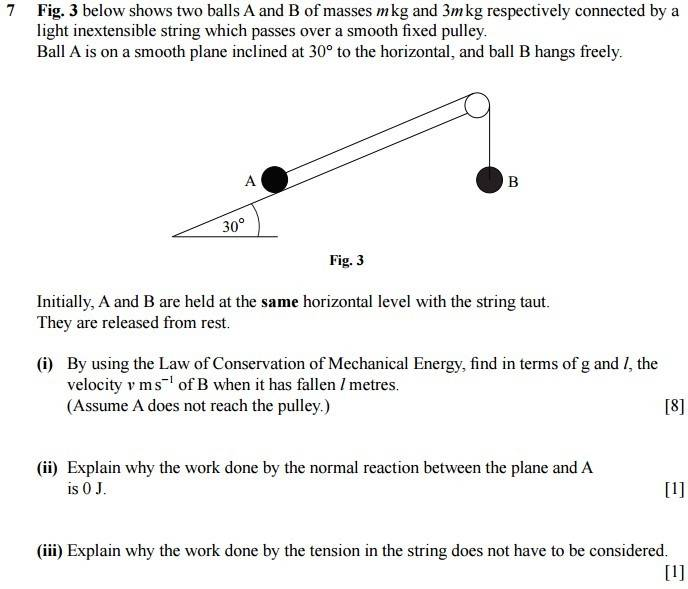# Finding the speed of an object on a pulley system

• TiernanW
In summary, the conversation discusses a problem involving the conservation of energy and the calculation of velocity using equations for potential and kinetic energy. The person asking the question made a mistake by using the incorrect mass in their calculation and the correct answer is achieved by using a mass of 3m instead of just m in the kinetic energy equation.

## Homework StatementPE = mgh
KE = 0.5mv^2
WD = F * s

## The Attempt at a Solution

Its part i. I understand the solution when you look at the whole system. You do not need to consider the tension in the string because they cancel out, but I want to be able to do it just considering B's KE and PE.

So I said that for B, the PE at the start is mgl and at the end it is considered 0. For its kinetic energy, at the start it is 0 and at the end it is 0.5mv^2. I also realize that it is subject to resistance from the tension in the string, so I resolved the forces to 3mg - T = 3ma (For B) and T - mgSin30 = ma (for A). From this I solved for T to get T = 9/8mg. Then I calculated the work done against tension as WD = -9/8mgl.

So then using the conservation of energy I said that 3mgl = 0.5mv^2 + 9/8mgl and for v I got (√15gl)/2. (g and l also in root)

The correct answer however is v = (√5gl)/2. (g and l also in root).

I know its easier to do it considering the whole system, but I want to do it this way, so what have I done wrong?

TiernanW said:

## Homework Statement

[ IMG]http://i.imgur.com/tsdVoFy.jpg[/PLAIN]

PE = mgh
KE = 0.5mv^2
WD = F * s

## The Attempt at a Solution

Its part i. I understand the solution when you look at the whole system. You do not need to consider the tension in the string because they cancel out, but I want to be able to do it just considering B's KE and PE.

So I said that for B, the PE at the start is mgl and at the end it is considered 0. For its kinetic energy, at the start it is 0 and at the end it is 0.5mv^2. I also realize that it is subject to resistance from the tension in the string, so I resolved the forces to 3mg - T = 3ma (For B) and T - mgSin30 = ma (for A). From this I solved for T to get T = 9/8mg. Then I calculated the work done against tension as WD = -9/8mgl.

So then using the conservation of energy I said that 3mgl = 0.5mv^2 + 9/8mgl and for v I got (√15gl)/2. (g and l also in root)

The correct answer however is v = (√5gl)/2. (g and l also in root).

I know its easier to do it considering the whole system, but I want to do it this way, so what have I done wrong?
You used a mass of m, not 3m, in the K.E.

#### Attachments

•TiernanW
SammyS said:
You used a mass of m, not 3m, in the K.E.
Shoot. Awkward haha. Thanks! :)